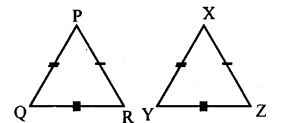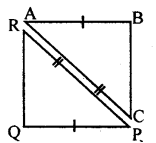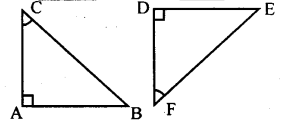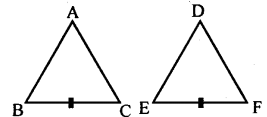# KSEEB Solutions for Class 8 Maths Chapter 11 Congruency of Triangles Ex 11.1

Students can Download Maths Chapter 11 Congruency of Triangles Ex 11.1 Questions and Answers, Notes Pdf, KSEEB Solutions for Class 8 Maths helps you to revise the complete Karnataka State Board Syllabus and score more marks in your examinations.

## Karnataka Board Class 8 Maths Chapter 11 Congruency of Triangles Ex 11.1

Question 1.
1. Identify the corresponding sides and corresponding angles in the following conruent triangles. and PR & XZ corresponding angles.Corresponding sides PQ & XY, QR & YZ, and PR & XZ corresponding angles.
∠P & ∠X, ∠Q & ∠Y, and ∠R and ∠Z.

2.Corresponding sides AB & QP, AC & RP and BC & RQ corresponding angles.
∠A & ∠P , ∠B & ∠Q and ∠C &∠R

Question 2.
Pair of the congruent triangle and incomplete statements related to them are given below. Observe the figures carefully and fill up the blanks
a. In the adjoining figure If ∠ L =∠F, then AB = _____ and BC = ______